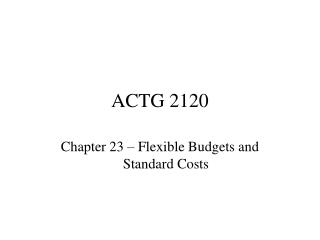DownloadDownload PresentationACTG 2120

# ACTG 2120

Download Presentation## ACTG 2120

- - - - - - - - - - - - - - - - - - - - - - - - - - - E N D - - - - - - - - - - - - - - - - - - - - - - - - - - -
##### Presentation Transcript

1. ACTG 2120 Chapter 23 – Flexible Budgets and Standard Costs

2. Flexible Budgets • Budgetary Control and Reporting • Fixed budget - budget prepared for one level • Budget prepared for 1,000 units • Costs \$10,000 + \$5 x • Budgeted Cost = \$15,000 • Suppose actual units prepared were 900 units costing \$14,700 • Did we beat budget?

3. Flexible Budgets • We did beat the fixed budget but that amount is really meaningless because we produced less units than the static budget • A flexible budget is a budget prepared for several different levels • A new budget must be produced for actual units produced • 900 units x \$5 = 4,500 + 10,000 = \$14,500 • Budget = \$14,500, Actual = \$14,700 • We are over budget by \$200.

4. Variance Analysis • Difference between actual and budget is called a VARIANCE. • For costs: • Variances are FAVORABLE if Actual < Standard • Variances are UNFAVORABLE if Actual > Standard • For revenues, it is the opposite: • Variances are UNFAVORABLE if Actual < Standard • Variances are FAVORABLE if Actual > Standard

5. Standard Cost Systems • Benchmark or norm used for planning and control purposes; a model or budget against which actual results are compared and evaluated. • Like predetermined OH rates • A budget broken down into one unit of good or service. • Developed for DM, DL, and OH (both variable and fixed) • Each category involves a price and quantity element

6. Standard Cost Systems • Types of standards • Ideal standards • Currently attainable standards • Who develops standard costs? • Engineers • Cost accountants • Managers • Workers

7. Standard Cost SystemsReasons for Using • Easier bookkeeping • Motivation • Planning • Control costs • Decision making • Performance evaluation

8. Standards • Revisions • Should they be revised? • How often? • Management by Exception • Examine only the variances that are “materially” different from budget • Examine the variances which are controllable by management

9. Direct Material Variances • Price Variance - difference in actual price paid per DM and standard price that should have been paid • Quantity Variance - difference in actual material used and material that should have been used • Causes

10. Direct Material Variances • AP x AQ SP x AQ purchased • |__________________| • Price Variance • SP x AQ used SP x SQ • |______________| • Quantity Variance

11. Standard Quantity Allowed • Standard Quantity per unit • X units produced • = Standard Quantity Allowed • Example: • 4 yards per sleeping bag • X 100 bags produced • = 400 yards of fabric allowed

12. Direct Labor Variances • Rate Variance - difference in rates actually paid and standard wage rates per hour • Time Variance - difference in actual hours worked and standard hours that should have been worked. • Causes

13. Direct Labor Variances • AR x AH SR x AH SR x SH • |__________________| |_____________| • Rate Variance Time Variance • |_________________________________| • Total Labor Variance

14. Responsibility for Variances • Materials price - Purchasing • Materials quantity - Production, Purchasing • Labor rate - Personnel, Production • Labor time - Production, Purchasing • BUT: • Must investigate the variance to see who is actually responsible

15. Overhead Variances • Total OH cost variance • Difference in actual OH and applied OH • Controllable • Volume variance • Causes

16. Overhead Variances • The standard price for the overhead is determined by the predetermined overhead rate. • This rate can be separated for variable and fixed overhead. • The rate is determined by choosing a level, the “denominator” level, at which a company thinks it will produce.

17. Predetermined OH Rates • Variable OH: \$4,500/900 = \$5 • \$5,000/1,000 = \$5 • \$5,500/1,100 = \$5 • It makes no difference which level of output we choose to set the variable OH rate. It is \$5 for all of the levels.

18. Predetermined OH Rates • Fixed OH: \$10,000/900 = \$11.11 • \$10,000/1,000 = \$10 • \$10,000/1,100 = \$9.09 • It DOES make a difference which level of output we choose to set the fixed OH rate. The OH rate varies from \$11.11 to \$9.09 based on the level of units we choose to set the rate. This chosen level is called the DENOMINATOR LEVEL.

19. Predetermined OH Rates • If we choose to use 900 units to set our OH rate, we will have the higher \$11.11 OH rate. • More overhead will be allocated than the other two levels. • We are more likely to have overapplied OH with a higher rate.

20. Controllable Variance • Difference in actual overhead and flexible budgeted overhead • Flexible budget = • Budgeted Variable OH rate x APPLIED activity (Actual units x budgeted variable OH quantity) • + Fixed budgeted OH

21. Volume Variance • Difference in • Budgeted total fixed overhead and • Predetermined fixed overhead rate x APPLIED activity (Actual units x Budgeted driver per unit) • Depends on denominator level chosen • Deemed an “uncontrollable” variance • Causes

22. Homework • Problems 23-1B*, 23-3B, BTN 23-7 • DUE WITH EXAM, FRIDAY, MAY 30